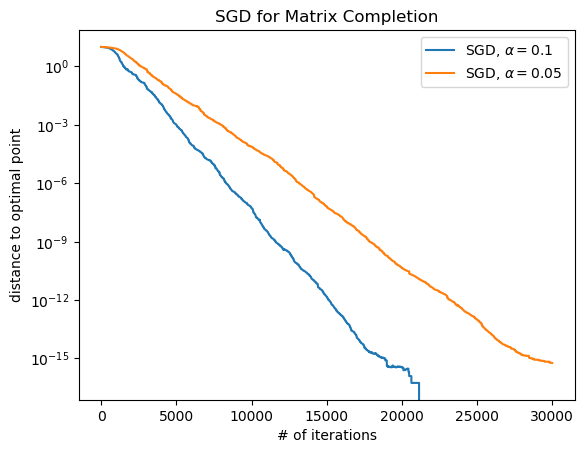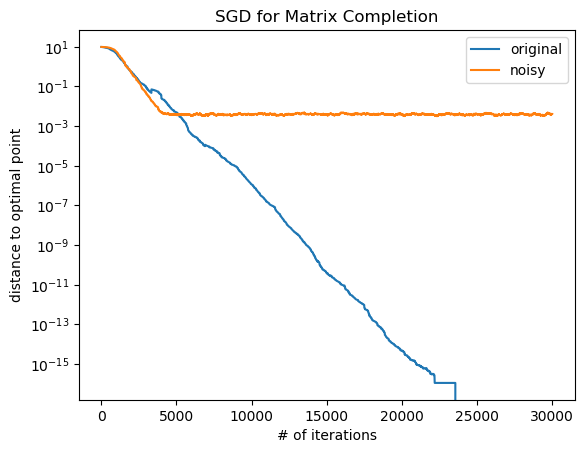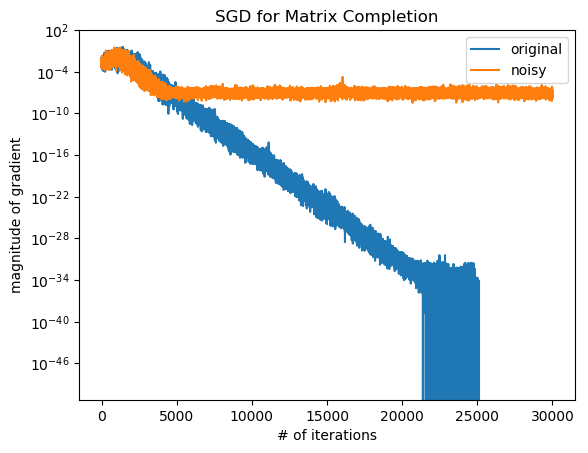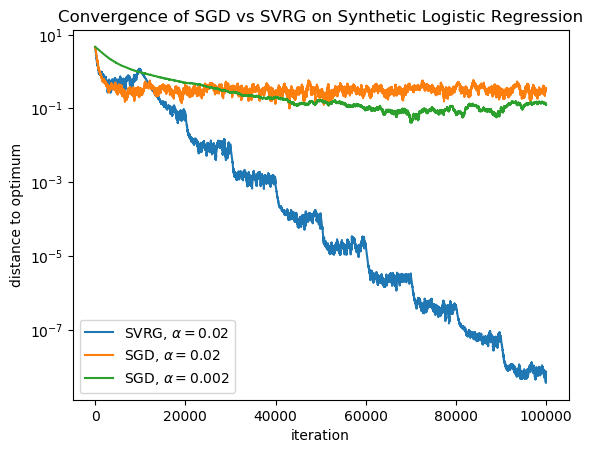# Part One: SGD for Rank-1 Matrix Completion¶

Goal here is to compute SGD for matrix completion: $$x_{t+1} = x_t - 2 \alpha (e_i^T x x^T e_j - e_i^T A e_j) (e_i e_j^T x + e_j e_i^T x).$$ First, we need to generate a matrix $A$ to try to recover at random.

In :
using PyPlot;
using StatsBase;
using LinearAlgebra;
using Random

Random.seed!(8675309);

┌ Info: Precompiling StatsBase [2913bbd2-ae8a-5f71-8c99-4fb6c76f3a91]

In :
n = 100;
xopt = randn(n);
A = xopt * xopt';

In :
# randomly sample the entries of A
K = 10000;
all_entries = [(i, j, A[i,j]) for i = 1:n for j = 1:n];
samples = sample(all_entries, K);

In :
# define SGD for matrix completion
function sgd_matrixcompletion(samples, x0, alpha, niters, xopt)
dists_to_optimum = zeros(niters);
x = copy(x0);
for k = 1:niters
(i, j, Aij) = rand(samples); # randomly choose a sample
xi = x[i];
xj = x[j];
x[i] -= 2 * alpha * (xi * xj - Aij) * xj;
x[j] -= 2 * alpha * (xi * xj - Aij) * xi;
dists_to_optimum[k] = min(norm(x - xopt), norm(x + xopt))
end
return (x, dists_to_optimum);
end

Out:
sgd_matrixcompletion (generic function with 1 method)
In :
x0 = randn(n) / 5;
(xsoln1, dto1) = sgd_matrixcompletion(samples, x0, 0.1, 3*K, xopt);
(xsoln2, dto2) = sgd_matrixcompletion(samples, x0, 0.05, 3*K, xopt);

In :
semilogy(dto1; label="SGD, \$\\alpha=0.1\$");
semilogy(dto2; label="SGD, \$\\alpha=0.05\$");
xlabel("# of iterations")
ylabel("distance to optimal point")
title("SGD for Matrix Completion")
legend();We actually are converging down to the point where numerical precision is having an affect on our algorithm!

This is puzzing. Why did we not converge to a noise ball?

## Hypotheses?¶

Was it the specific way we did the sampling that caused this effect?

What if we sampled in a different way, where the samples are still unbiased in expectation, but have a little extra variance? For example:

$$x_{t+1} = x_t - 2 \alpha (e_i^T x x^T e_j - e_i^T A e_j + \tilde z_t) (e_i e_j^T x + e_j e_i^T x).$$

where $\tilde z_t ~ N(0, \sigma^2)$ is a normally distributed random variable.

In :
sigma = 0.001;

In :
# define SGD for this new noisy matrix completion
function sgd_noisy_matrixcompletion(samples, x0, alpha, niters, xopt, sigma)
dists_to_optimum = zeros(niters);
x = copy(x0);
for k = 1:niters
(i, j, Aij) = rand(samples); # randomly choose a sample
xi = x[i];
xj = x[j];
z = sigma * randn();
x[i] -= 2 * alpha * (xi * xj - Aij + z) * xj;
x[j] -= 2 * alpha * (xi * xj - Aij + z) * xi;
dists_to_optimum[k] = min(norm(x - xopt), norm(x + xopt))
end
return (x, dists_to_optimum);
end

Out:
sgd_noisy_matrixcompletion (generic function with 1 method)
In :
x0 = randn(n) / 5;
(xsoln, dto) = sgd_matrixcompletion(samples, x0, 0.1, 3*K, xopt);
(xsoln_noisy, dto_noisy) = sgd_noisy_matrixcompletion(samples, x0, 0.1, 3*K, xopt, sigma);

In :
semilogy(dto; label = "original");
semilogy(dto_noisy; label = "noisy");
xlabel("# of iterations");
ylabel("distance to optimal point");
title("SGD for Matrix Completion");
legend();When we add even a small amount of noise, now it converges to a noise ball again.

So it is something about the way we sampled that caused this effect!

Does it have something to do with the variance of the gradient estimator?

In :
# define versions of the functions that estimate the variance by reporting the second moment of the gradient

function sgd_matrixcompletion_ve(samples, x0, alpha, niters, xopt, gssize)
dists_to_optimum = zeros(niters);
x = copy(x0);
for k = 1:niters
(i, j, Aij) = rand(samples); # randomly choose a sample
xi = x[i];
xj = x[j];
x[i] -= 2 * alpha * (xi * xj - Aij) * xj;
x[j] -= 2 * alpha * (xi * xj - Aij) * xi;
dists_to_optimum[k] = min(norm(x - xopt), norm(x + xopt));
gm = 0.0;
for gi = 1:gssize
(i, j, Aij) = rand(samples); # randomly choose a sample
xi = x[i];
xj = x[j];
gm += (2 * alpha * (xi * xj - Aij))^2 * (xi^2 + xj ^2);
end
end
end

function sgd_noisy_matrixcompletion_ve(samples, x0, alpha, niters, xopt, sigma, gssize)
dists_to_optimum = zeros(niters);
x = copy(x0);
for k = 1:niters
(i, j, Aij) = rand(samples); # randomly choose a sample
xi = x[i];
xj = x[j];
z = sigma * randn();
x[i] -= 2 * alpha * (xi * xj - Aij + z) * xj;
x[j] -= 2 * alpha * (xi * xj - Aij + z) * xi;
dists_to_optimum[k] = min(norm(x - xopt), norm(x + xopt));
gm = 0.0;
for gi = 1:gssize
(i, j, Aij) = rand(samples); # randomly choose a sample
xi = x[i];
xj = x[j];
z = sigma * randn();
gm += (2 * alpha * (xi * xj - Aij + z))^2 * (xi^2 + xj ^2);
end
end
end

Out:
sgd_noisy_matrixcompletion_ve (generic function with 1 method)
In :
x0 = randn(n) / 5;
(xsoln, dto, gm) = sgd_matrixcompletion_ve(samples, x0, 0.1, 3*K, xopt, 10);
(xsoln_noisy, dto_noisy, gm_noisy) = sgd_noisy_matrixcompletion_ve(samples, x0, 0.1, 3*K, xopt, sigma, 10);

In :
semilogy(gm; label = "original");
semilogy(gm_noisy; label = "noisy");
xlabel("# of iterations");
title("SGD for Matrix Completion");
legend();So what's actually happening here?

The variance of the estimator is going to zero as the algorithm converges!

This is different from how it works for SGD for most problems, where the variance is nonzero even at the optimal point.

# Part Two: Stochastic Variance-Reduced Gradient (SVRG)¶

In :
Random.seed!(8675309);

In :
# generate a naive bayes model
d = 10;
N = 10000;
wtrue = randn(d);
X = randn(N, d);
y = (1 ./ (1 .+ exp.(-X * wtrue)) .>= rand(N)) * 2 .- 1;

In :
function sgd(w0, alpha, X, y, niters, wopt)
w = w0
(N, d) = size(X)
dist_to_optimum = zeros(niters)
for k = 1:niters
i = rand(1:N)
xi = X[i,:];
yi = y[i];
w = w + alpha * xi * yi ./ (1 .+ exp.(yi * dot(xi, w)));
dist_to_optimum[k] = norm(w - wopt);
end
return (w, dist_to_optimum);
end

function svrg(w0, alpha, X, y, niters, nepochs, wopt)
w = w0
(N, d) = size(X)
dist_to_optimum = zeros(niters * nepochs)
for epi = 1:nepochs
wtilde = w;
gtilde = zeros(d);
for i = 1:N
xi = X[i,:];
yi = y[i];
gtilde += xi * yi ./ (1 .+ exp.(yi * dot(xi, wtilde)));
end
gtilde /= N;
for k = 1:niters
i = rand(1:N)
xi = X[i,:];
yi = y[i];
w = w + alpha * (xi * yi ./ (1 .+ exp.(yi * dot(xi, w))) - xi * yi ./ (1 .+ exp.(yi * dot(xi, wtilde))) + gtilde);
dist_to_optimum[k + (epi-1)*niters] = norm(w - wopt);
end
end
return (w, dist_to_optimum);
end

Out:
svrg (generic function with 1 method)
In :
# initial random weights
w0 = randn(d);

In :
# solve the problem using SVRG to get the optimum, which we'll use later.
(wopt, dto_opt) = svrg(w0, 0.02, X, y, 10000, 100, wtrue);
sgd(w0, 0.02, X, y, 5000 * 20, wopt);

In :
@time (w, dto) = svrg(w0, 0.02, X, y, 10000, 10, wopt);
@time (w, dto2) = sgd(w0, 0.02, X, y, 5000 * 20, wopt);
@time (w, dto3) = sgd(w0, 0.002, X, y, 5000 * 20, wopt);

  0.103363 seconds (1.40 M allocations: 214.389 MiB, 5.79% gc time)
0.041457 seconds (600.01 k allocations: 92.316 MiB, 4.68% gc time)
0.041388 seconds (600.01 k allocations: 92.316 MiB, 4.79% gc time)

In :
semilogy(dto; label="SVRG, \$\\alpha=0.02\$");
semilogy(dto2; label="SGD, \$\\alpha=0.02\$");
semilogy(dto3; label="SGD, \$\\alpha=0.002\$");

title("Convergence of SGD vs SVRG on Synthetic Logistic Regression");
ylabel("distance to optimum");
xlabel("iteration");
legend();In [ ]: